# Ultrasonic distance sensor

In times of Corona, it is very important to keep distance

BeginnerWork in progress1 hour250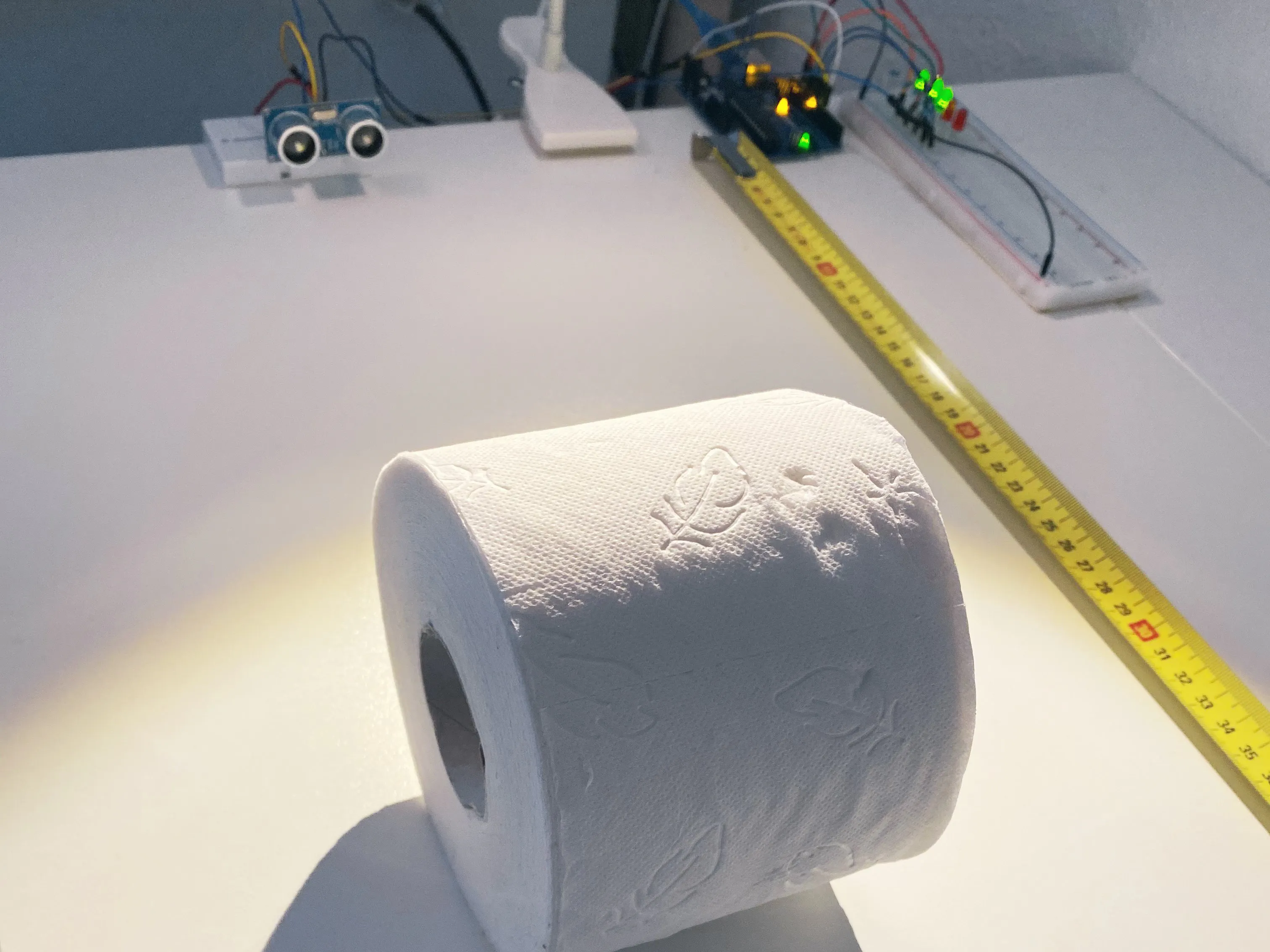## Things used in this project

### Hardware componentsArduino UNO & Genuino UNO
×1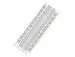×1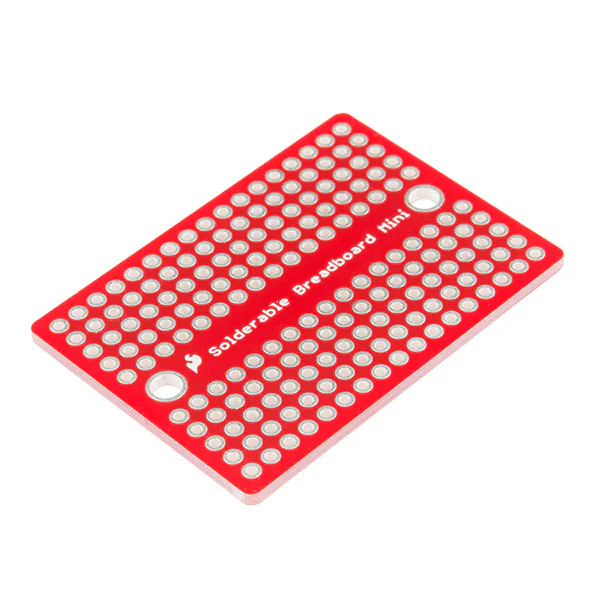×1LED (generic) 2x Red, 3xGreen
×1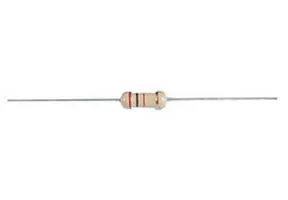Resistor 220 ohm
×1Jumper wires (generic)
×10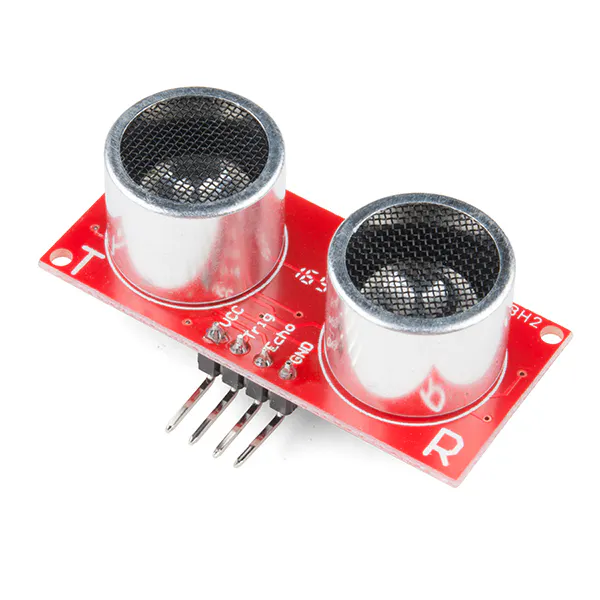SparkFun Ultrasonic Sensor - HC-SR04
×1

## Schematics

### Sketch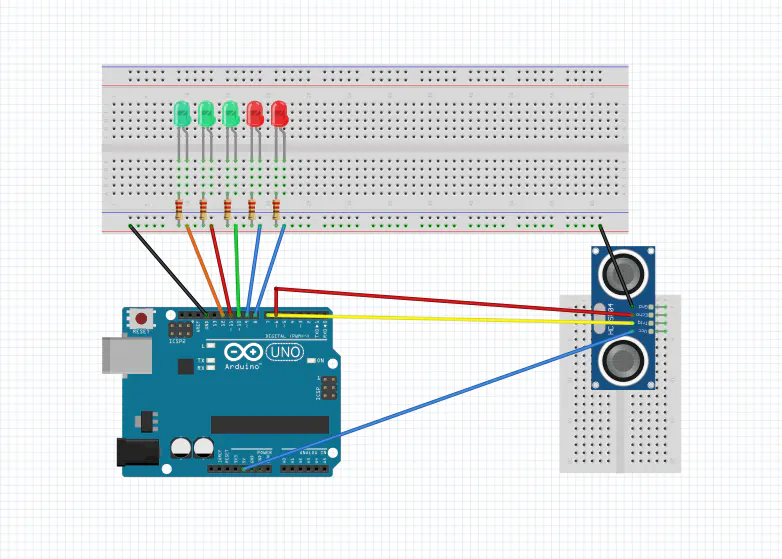## Code

### Untitled file

C/C++
```int trigger = 7;
int echo = 6;
long Time = 0;
long  distance = 0;
int G1 = 12;
int G2 = 11; //
int G3 = 10; //
int R4 = 9; //
int R5 = 8; //
int Abstand = 90;
int Zeit = 50;

void setup ()
{
Serial.begin(9600);
pinMode(trigger, OUTPUT);
pinMode(echo, INPUT);
pinMode(G1, OUTPUT);
pinMode(G2, OUTPUT);
pinMode(G3, OUTPUT);
pinMode(R4, OUTPUT);
pinMode(R5, OUTPUT);
}

void loop()
{
digitalWrite(trigger, LOW);
delay(5);
digitalWrite(trigger, HIGH);
delay(10);
digitalWrite(trigger, LOW);
Time = pulseIn(echo, HIGH);
distance = (Time/2) * 0.03432; //

if (distance >= 500 || distance <= 0)
{Serial.println("Kein Messwert");}
else
{Serial.print(distance);
Serial.println("cm");}

delay(50);

if (distance <= Abstand)
{digitalWrite(G1, HIGH);
}

else
{
digitalWrite(G1, LOW);
}

if (distance <= Abstand - 50)
{ digitalWrite(G2, HIGH);}

else
{digitalWrite(G2, LOW);}

if (distance <= Abstand - 60)
{digitalWrite(G3, HIGH);}

else
{ digitalWrite(G3, LOW);}

if (distance <= Abstand - 70)
{digitalWrite(R4, HIGH);}

else
{digitalWrite(R4, LOW);}

if (distance <= Abstand - 80)
{digitalWrite(R5, HIGH);}

else
{digitalWrite(R5, LOW);

}

delay(5);

}
```

### Video

Vala
`No preview (download only).`

## Credits

### Joshua Fieger

0 projects • 0 followers